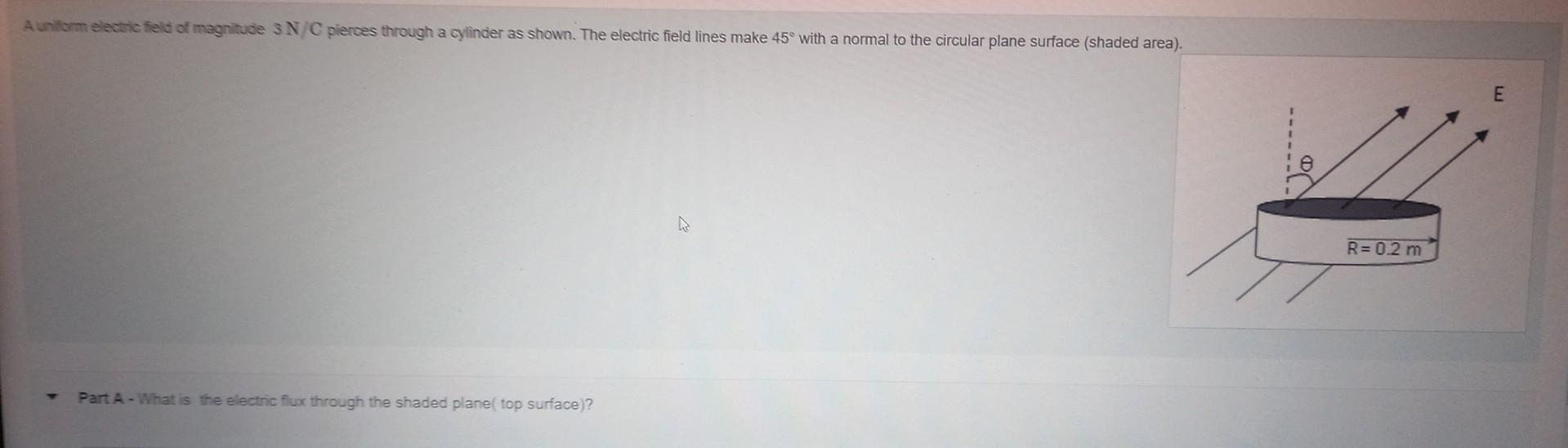# Question   What is the electric flux through the shaded plane(top surface)? r=0.2 E=3N/c 45° Part A - What is the electric fiux through the shaded plane( top surface)?What is the electric flux through the shaded plane(top surface)?
r=0.2
E=3N/c
45°

Transcribed Image Text: Part A - What is the electric fiux through the shaded plane( top surface)?
More
Transcribed Image Text: Part A - What is the electric fiux through the shaded plane( top surface)?&#12304;General guidance&#12305;The answer provided below has been developed in a clear step by step manner.Step1/1Electric flux through a surface is given by&#981;=EAcos&#8289;(&#952;)&#160;Where E = magnitude of electric field passing through surface = 3N/C A = surface area = $$\mathrm{π{R}^{{2}}={3.14}×{\left({0.2}\right)}^{{2}}{m}^{{2}}={0.125}{m}^{{2}}}$$&#160;&#952;&#160; = angle between normal to surface and el ... See the full answer